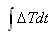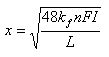# Stefan Formula

Some of the first studies of freeze/thaw depth were made by Josef Stefan in 1889, in connection with ice formation and melting in the Polar oceans (Paynter, not date given). In this formula it is assumed that the latent heat of soil moisture is the only heat that must be removed when freezing the soil. Thus, thermal energy stored as volumetric heat and released as soil-temperatures drop to and below freezing is not considered. Because volumetric heat is neglected, the Stefan Formula tends to overestimate frost depth in temperate zones (Paynter, no date given). The latent heat supplied by the soil moisture as it freezes a depth dx in time dt = rate at which heat is conducted to the ground surface. This is illustrated in the sketch below:

Heat removal process can be represented by(heat released by freezing a layer of soil dx thick in time dt)(heat conducted through frozen layer)

and Q1 = Q2 soby integrating and solving for x,is in units of °F • hr and is called surface freezing index. The freezing index is normally expressed as °F • days. Thus, rewrite the equation and add an “n” factor which results in the Stefan formula: